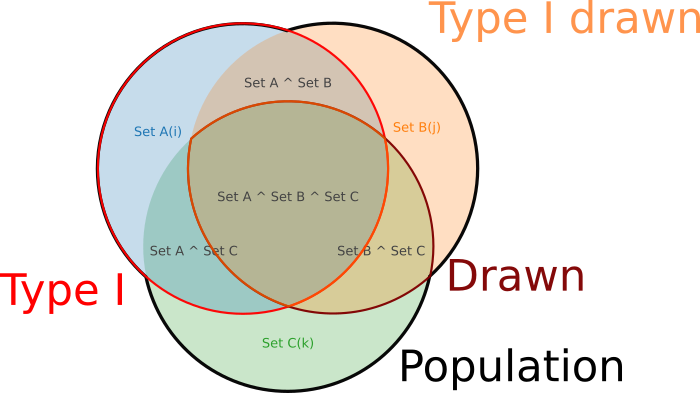## DynaVenn - Find most significant overlap between subsets of gene lists

The main goal of DynaVenn is to find the most significant overlap between subsets of gene lists, starting from the top of the lists. We define the sets $A$, $B$ and $C$ with $|A| = l$, $|B| = m$ and $|C| = n$. We are interested in finding the indices $i, j, k$ ($1 \leq i \leq l$, $1 \leq j \leq m$ and $1 \leq k \leq n$) so that the overlap between the ordered lists $A[1:l], B[1:m], C[1:n]$ is maximized.

### Mathematical Background

#### Hypergeometric density

$p(k, M, n, N) = \frac{\binom{n}{k} \binom{M-n}{N-k}}{\binom{M}{N}}$

with the binomial coefficients defined as

$\binom{n}{k} = \frac{n!}{k!(n-k)!}$

It models drawing object from a bin. $M$ is the total number of objects, $n$ the number of white balls, $N$ the number of drawn balls and $k$ the number of white balls among the drawn balls. Applied to the overlap of genes, we get the following formula:

$1 - cdf(k, M, n, N) = 1 - \sum\limits_{i=0}^n \frac{\binom{n}{k} \binom{M-n}{N-k}}{\binom{M}{N}}$

##### Two gene sets
In the context of two lists, the values are chosen as with $k = overlap(A[:i], B[:j])$, $M = A \cup B$, $n = i$ and $N = j$.
##### Three gene sets
The test with three gene lists works equivalently, with $k = \lvert A[1:i]\cap B[1:j] \cap C[1:k]\rvert - 1$, $M = \lvert A \cup B \cup C\rvert$, $n = i$ and $N = \lvert B[1:j] \cap C[1:k]\rvert$.#### Backtracking

A backtracking step is carried out similar to the backtracking in the dynamic programming solution of sequence alignments. The backtracking procedures expands into two directions: starting from the position of the smallest p-value, the path goes into the direction of the empty and on the other side in the direction of the full sets.

For each position in the matrix (i,j) that contains the p-value for the first i and j elements, the matrix positions (i-1,j) and (i,j-1) are considered. Thereby, in each step it is decided whether an element of the first or the second list has to be added in order to come to the optimal p-value. In the case of three lists, at position (i,j,k) in the cube, the positions (i-1,j,k), (i,j-1,k) and (i,j,k-1) are considered. In each case, the result of the algorithm is the sequence of items of the two or three sets that have to be added in order to find the Venn diagram with the most significant overlap.